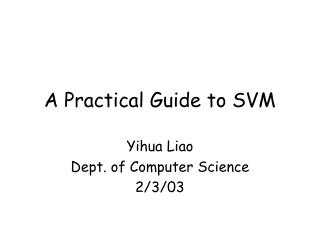DownloadDownload PresentationA Practical Guide to SVM

A Practical Guide to SVM

Download PresentationA Practical Guide to SVM

- - - - - - - - - - - - - - - - - - - - - - - - - - - E N D - - - - - - - - - - - - - - - - - - - - - - - - - - -
Presentation Transcript

1. A Practical Guide to SVM Yihua Liao Dept. of Computer Science 2/3/03

2. Outline • Support vector machine basics • GIST • LIBSVM (SVMLight)

3. Classification problems • Given: n training pairs, (<xi>, yi), where <xi>=(xi1, xi2,…,xil) is an input vector, and yi=+1/-1, corresponding classification H+ /H- • Out: A label y for a new vector x

4. Support vector machines Goal: to find discriminator That maximize the margins

5. A little math • Primal problem • Decision function

6. Example • Functional classifications of Yeast genes based on DNA microarray expression data. • Training dataset • genes that are known to have the same Function f • genes that are known to have a different function than f

7. Gist • http://microarray.cpmc.columbia.edu/gist/ • Developed by William Stafford Noble etc. • Contains tools for SVM classification, feature selection and kernel principal components analysis. • Linux/Solaris. Installation is straightforward.

8. Data files • Sample.mtx(tab-delimited, same for testing) gene alpha_0X alpha_7X alpha_14X alpha_21X … YMR300C -0.1 0.82 0.25 -0.51 … YAL003W 0.01 -0.56 0.25 -0.17 … YAL010C -0.2 -0.01 -0.01 -0.36 … … • Sample.labels gene Respiration_chain_complexes.mipsfc YMR300C -1 YAL003W 1 YAL010C -1

9. Usage of Gist • \$compute-weights -train sample.mtx -class sample.labels > sample.weights • \$classify -train sample.mtx -learned sample.weights -test test.mtx > test.predict • \$score-svm-results -test test.labelstest.predict sample.weights

10. Test.predict # Generated by classify # Gist, version 2.0 …. gene classification discriminant YKL197C -1 -3.349 YGL022W -1 -4.682 YLR069C -1 -2.799 YJR121W 1 0.7072

11. Output of score-svm-results Number of training examples: 1644 (24 positive, 1620 negative) Number of support vectors: 60 (14 positive, 46 negative) 3.65% Training results: FP=0 FN=3 TP=21 TN=1620 Training ROC: 0.99874 Test results: FP=12 FN=1 TP=9 TN=801 Test ROC: 0.99397

12. Parameters • compute-weights • -power <value> • -radial -widthfactor <value> • -posconstraint <value> • -negconstraint <value> …

13. Rules of thumb • Radial basis kernel usually performs better. • Scale your data. scale each attribute to [0,1] or [-1,+1] to avoid over-fitting. • Try different penalty parameters C for two classes in case of unbalanced data.

14. LIBSVM • http://www.csie.ntu.edu.tw/~cjlin/libsvm/ • Developed by Chih-Jen Lin etc. • Tools for (multi-class) SV classification and regression. • C++/Java/Python/Matlab/Perl • Linux/UNIX/Windows • SMO implementation, fast!!!

15. Data files for LIBSVM • Training.dat +1 1:0.708333 2:1 3:1 4:-0.320755 -1 1:0.583333 2:-1 4:-0.603774 5:1 +1 1:0.166667 2:1 3:-0.333333 4:-0.433962 -1 1:0.458333 2:1 3:1 4:-0.358491 5:0.374429 … • Testing.dat

16. Usage of LIBSVM • \$svm-train -c 10 -w1 1 -w-1 5 Train.dat My.model - train classifier with penalty 10 for class 1 and penalty 50 for class –1, RBK • \$svm-predict Test.dat My.model My.out • \$svm-scaleTrain_Test.dat > Scaled.dat

17. Output of LIBSVM • Svm-train optimization finished, #iter = 219 nu = 0.431030 obj = -100.877286, rho = 0.424632 nSV = 132, nBSV = 107 Total nSV = 132

18. Output of LIBSVM • Svm-predict Accuracy = 86.6667% (234/270) (classification) Mean squared error = 0.533333 (regression) Squared correlation coefficient = 0.532639 (regression) • Calculate FP, FN, TP, TN from My.out